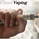12932 views
12932
BB Support + Histo mode
-------------------------------
Code: http://pastebin.com/4QKdCEs7

Show enclosing BB

Show Insync as Histo:

v02 - Configurable levels
---------------------------------

Small update to allow configuring the 95/75/25/5 levels.

Latest source code: http://pastebin.com/fZ4PmemR

v01 - orginal description
---------------------------------

Insync Index, by Norm North, is a consensus indicator. It uses RSI , MACD , MFI , DPO , ROC , Stoch , CCI and %B to calculate a composite signal. Basically, this index shows that when a majority of underlying indicators is in sync, a turning point is near.

There are couple of ways to use this indicator.
- Buy when crossing up 5, sell when crossing down 95.
- Market is typically bullish when index is above 50, bearish when below 50. This can be a great confirmation signal for price action + trend lines .

Also, since this is typical oscillator, look for divergences between price and index.

Levels 75/25 are early warning levels. Note that, index > 75 (and less than 95) should be considered very bullish and index below 25 (but above 5) as very bearish . Levels 95/5 are equivalent to traditional OB/OS levels.

The various values of the underlying components can be tuned via options page. I have also provided an option to color bars based on the index value.

List of my free indicators: http://bit.ly/1LQaPK8
List of my app-store indicators: http://blog.tradingview.com/?p=970
(Support doc: http://bit.ly/lazybeardoc)
```//
// @author LazyBear
// List of all my indicators: http://bit.ly/1LQaPK8
//
study(title = "Insync Index [LazyBear]", shorttitle="II_LB")
src=close
div = input(10000, title="EMO Divisor", minval=1)
emoLength = input(14, minval=1, title="EMO length")
fastLength = input(12, minval=1, title="MACD Fast EMA Length")
slowLength=input(26,minval=1, title="MACD Slow EMA Length")
signalLength=input(9,minval=1, title="MACD Signal Length")
mfiLength = input(20, minval=1, title="MFI Length")

calc_emo() => sma(div * change(hl2) * (high - low) / volume, emoLength)
calc_macd(source) =>
fastMA = ema(source, fastLength)
slowMA = ema(source, slowLength)
fastMA - slowMA

calc_mfi(length) =>
src = hlc3
upper = sum(volume * (change(src) <= 0 ? 0 : src), length)
lower = sum(volume * (change(src) >= 0 ? 0 : src), length)
mf = rsi(upper, lower)
mf

calc_dpo(period_) =>
isCentered = false
barsback = period_/2 + 1
ma = sma(close, period_)
dpo = isCentered ? close[barsback] - ma : close - ma[barsback]
dpo

calc_roc(source, length) =>
roc = 100 * (source - source[length])/source[length]
roc

calc_stochD(length, smoothD, smoothK) =>
k = sma(stoch(close, high, low, length), smoothK)
d = sma(k, smoothD)
d

calc_stochK(length, smoothD, smoothK) =>
k = sma(stoch(close, high, low, length), smoothK)
//d = sma(k, smoothD)
k

lengthBB=input(20, title="BB Length"), multBB=input(2.0, title="BB Multiplier")
lengthCCI=input(14, title="CCI Length")
dpoLength=input(18, title="DPO Length")
lengthROC=input(10, title="ROC Length")
lengthRSI=input(14, title="RSI Length")
lengthStoch=input(14, title="Stoch Length"),lengthD=input(3, title="Stoch D Length"), lengthK=input(1, title="Stoch K Length")
lengthSMA=input(10, title="MA Length")

bolinslb=sma( src,lengthBB ) - multBB * ( stdev( src,lengthBB ) )
bolinsub=sma( src,lengthBB ) + multBB * ( stdev( src,lengthBB ) )
bolins2= (src- bolinslb ) / ( bolinsub - bolinslb )
bolinsll=( bolins2 < 0.05 ? -5 : ( bolins2 > 0.95 ? 5 : 0 ) )
cciins= ( cci(src, lengthCCI) > 100 ? 5 :  ( cci(src, lengthCCI ) < -100 ? -5 : 0 ) )
emvins2= calc_emo() - sma( calc_emo(),lengthSMA)
emvinsb= ( emvins2 < 0 ? ( sma( calc_emo() ,lengthSMA ) < 0 ? -5 : 0 ) : 0 )
emvinss= ( emvins2 > 0 ? ( sma( calc_emo() ,lengthSMA ) > 0 ? 5  : 0 ) : 0 )
macdins2= calc_macd( src) - sma( calc_macd( src) ,lengthSMA )
macdinsb= ( macdins2 < 0 ? ( sma( calc_macd( src),lengthSMA ) < 0 ? -5 : 0 ) : 0 )
macdinss=( macdins2 > 0 ? ( sma( calc_macd( src),lengthSMA) > 0 ? 5 : 0 ) : 0 )
mfiins=( calc_mfi( mfiLength ) > 80 ? 5 : ( calc_mfi( mfiLength ) < 20 ? -5 : 0 ) )
pdoins2=calc_dpo( dpoLength ) - sma( calc_dpo( dpoLength ),lengthSMA )
pdoinsb=( pdoins2 < 0 ? ( sma( calc_dpo( dpoLength ),lengthSMA) < 0 ? -5 : 0 ) :0 )
pdoinss=( pdoins2 > 0 ? ( sma( calc_dpo( dpoLength ),lengthSMA) > 0 ? 5 : 0 ) :0 )
rocins2=calc_roc( src,lengthROC  ) - sma( calc_roc( src,lengthROC ),lengthSMA  )
rocinsb=( rocins2 < 0 ? ( sma( calc_roc( src,lengthROC ),lengthSMA  ) < 0 ? -5 : 0 ) : 0 )
rocinss = ( rocins2 > 0 ? ( sma( calc_roc( src,lengthROC ),lengthSMA ) > 0 ? 5 : 0 ) : 0 )
rsiins= ( rsi(src, lengthRSI ) > 70 ? 5  : ( rsi(src, lengthRSI )  < 30 ? -5 : 0 ) )

stopdins=( calc_stochD(lengthStoch,lengthD,lengthK ) > 80 ? 5 : ( calc_stochD(lengthStoch,lengthD, lengthK ) < 20 ? -5 : 0 ) )
stopkins=( calc_stochK(lengthStoch,lengthD,lengthK) > 80 ? 5 : ( calc_stochK(lengthStoch,lengthD, lengthK ) < 20 ? -5 : 0 ) )

iidx = 50 + cciins + bolinsll + rsiins + stopkins + stopdins + mfiins + emvinsb + emvinss + rocinss + rocinsb + nz(pdoinss) + nz(pdoinsb ) + macdinss + macdinsb
ml=plot(50, color=gray, title="Line50")
ll=plot(5, color= green, title="Line5")
ul=plot(95, color = red, title="Line95")

plot(25, color= green, style=3, title="Line25")
plot(75, color = red, style=3, title="Line75")

fill(ml, ll, color=red)
fill(ml, ul, color=green)

il=plot(iidx, color=maroon, linewidth=2, title="InsyncIndex")
fill(ml,il,black)

bc = iidx >= 50 ? (iidx >= 95 ? #336600 : iidx >= 75 ? #33CC00 : #00FF00) :
(iidx <= 5 ? #990000 : iidx <= 25? #CC3300 :  #CC9900)

ebc = input(false, title="Enable Barcolors")
barcolor(ebc?bc:na)```
List of my free indicators: http://bit.ly/1LQaPK8
List of my indicators at Appstore: http://blog.tradingview.com/?p=970Came across this indicator by chance, been giving it a thorough test run, and damn!...this thing is deadly accurate (would seriously pay for this indicator to have it on my MT5). Thanks LazyBear...andreas73
Here's an updated source that can be shown as Histo: http://pastebin.com/4QKdCEs7

Sample chart: